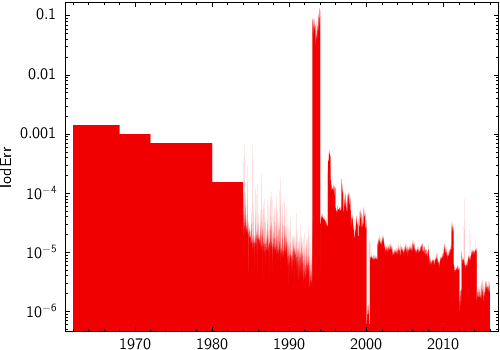Next Previous Up Contents
Next: quantile
Up: Layer Types
Previous: grid

#### 8.3.29 `fill`

If a two-dimensional dataset represents a single-valued function, this plotter will fill the area underneath the function's curve with a solid colour. Parts of the surface which would only be partially covered (because of rapid function variation within the width of a single pixel) are represented using appropriate alpha-blending. The filled area may alternatively be that above the curve or to its left or right.

One example of its use is to reconstruct the appearance of a histogram plot from a set of histogram bins. For X,Y data which is not single-valued, the result may not be very useful.

Usage Overview:

```   layerN=fill colorN=<rrggbb>|red|blue|... transparencyN=0..1
horizontalN=true|false positiveN=true|false <pos-coord-paramsN>
inN=<table> ifmtN=<in-format> istreamN=true|false icmdN=<cmds>
```

All the parameters listed here affect only the relevant layer, identified by the suffix `N`.

Positional Coordinate Parameters:
The positional coordinates `<pos-coord-paramsN>` give a position for each row of the input table. Their form depends on the plot geometry, i.e. which plotting command is used. For a plane plot (`plot2plane`) the parameters would be `xN` and `yN`. The coordinate parameter values are in all cases strings interpreted as numeric expressions based on column names. These can be column names, fixed values or algebraic expressions as described in Section 10.

Example:```   stilts plot2time layer1=fill in1=iers.fits t1=decYear y1=lodErr ylog=true
texttype=latex fontsize=16```

`colorN = <rrggbb>|red|blue|...`       (Color)
The color of plotted data, given by name or as a hexadecimal RGB value.

The standard plotting colour names are `red`, `blue`, `green`, `grey`, `magenta`, `cyan`, `orange`, `pink`, `yellow`, `black`, `light_grey`, `white`. However, many other common colour names (too many to list here) are also understood. The list currently contains those colour names understood by most web browsers, from `AliceBlue` to `YellowGreen`, listed e.g. in the Extended color keywords section of the CSS3 standard.

Alternatively, a six-digit hexadecimal number RRGGBB may be supplied, optionally prefixed by "`#`" or "`0x`", giving red, green and blue intensities, e.g. "`ff00ff`", "`#ff00ff`" or "`0xff00ff`" for magenta.

[Default: `red`]

`horizontalN = true|false`       (Boolean)
Determines whether the filling is vertical (suitable for functions of the horizontal variable), or horizontal (suitable for functions of the vertical variable). If false, the fill is vertical (to the X axis), and if true, the fill is horizontal (to the Y axis).

[Default: `false`]

`icmdN = <cmds>`       (ProcessingStep[])
Specifies processing to be performed on the layer N input table as specified by parameter `inN`. The value of this parameter is one or more of the filter commands described in Section 6.1. If more than one is given, they must be separated by semicolon characters (";"). This parameter can be repeated multiple times on the same command line to build up a list of processing steps. The sequence of commands given in this way defines the processing pipeline which is performed on the table.

Commands may alteratively be supplied in an external file, by using the indirection character '@'. Thus a value of "`@filename`" causes the file `filename` to be read for a list of filter commands to execute. The commands in the file may be separated by newline characters and/or semicolons, and lines which are blank or which start with a '`#`' character are ignored.

`ifmtN = <in-format>`       (String)
Specifies the format of the input table as specified by parameter `inN`. The known formats are listed in Section 5.1.1. This flag can be used if you know what format your table is in. If it has the special value `(auto)` (the default), then an attempt will be made to detect the format of the table automatically. This cannot always be done correctly however, in which case the program will exit with an error explaining which formats were attempted. This parameter is ignored for scheme-specified tables.

[Default: `(auto)`]

`inN = <table>`       (StarTable)
The location of the input table. This may take one of the following forms:
• A filename.
• A URL.
• The special value "`-`", meaning standard input. In this case the input format must be given explicitly using the `ifmtN` parameter. Note that not all formats can be streamed in this way.
• A scheme specification of the form `:<scheme-name>:<scheme-args>`.
• A system command line with either a "`<`" character at the start, or a "`|`" character at the end ("`<syscmd`" or "`syscmd|`"). This executes the given pipeline and reads from its standard output. This will probably only work on unix-like systems.
In any case, compressed data in one of the supported compression formats (gzip, Unix compress or bzip2) will be decompressed transparently.
`istreamN = true|false`       (Boolean)
If set true, the input table specified by the `inN` parameter will be read as a stream. It is necessary to give the `ifmtN` parameter in this case. Depending on the required operations and processing mode, this may cause the read to fail (sometimes it is necessary to read the table more than once). It is not normally necessary to set this flag; in most cases the data will be streamed automatically if that is the best thing to do. However it can sometimes result in less resource usage when processing large files in certain formats (such as VOTable). This parameter is ignored for scheme-specified tables.

[Default: `false`]

`positiveN = true|false`       (Boolean)
Determines the directional sense of the filling. If false, the fill is between the data points and negative infinity along the relevant axis (e.g. down from the data points to the bottom of the plot). If true, the fill is in the other direction.

[Default: `false`]

`transparencyN = 0..1`       (Double)
Transparency with which components are plotted, in the range 0 (opaque) to 1 (invisible). The value is 1-alpha.

[Default: `0`]

Next Previous Up Contents
Next: quantile
Up: Layer Types
Previous: grid

STILTS - Starlink Tables Infrastructure Library Tool Set